In :
#Import the required modules for vectors and matrix operations, data generation
import numpy as np
#Import the required modules for plot creation:
import matplotlib.pyplot as plt
#import the required modules for TimeSeries data generation:
import statsmodels.api as sm
#Import the required modules for test statistic calculation:
import statsmodels.stats as sm_stat
#Import the required modules for model estimation:
import statsmodels.tsa as smt
#Import formula specification
import statsmodels.formula.api as smf
# Import pandas dataset
import pandas as pd


We will also need some custom functions to make ploting easier:

In :
def tsdisplay(y, figsize = (14, 8), title = "", lags = 20):
tmp_data = pd.Series(y)
fig = plt.figure(figsize = figsize)
#Plot the time series
tmp_data.plot(ax = fig.add_subplot(311), title = "$Time\ Series\ " + title + "$", legend = False)
#Plot the ACF:
sm.graphics.tsa.plot_acf(tmp_data, lags = lags, zero = False, ax = fig.add_subplot(323))
plt.xticks(np.arange(1,  lags + 1, 1.0))
#Plot the PACF:
sm.graphics.tsa.plot_pacf(tmp_data, lags = lags, zero = False, ax = fig.add_subplot(324))
plt.xticks(np.arange(1,  lags + 1, 1.0))
#Plot the QQ plot of the data:
plt.title("QQ Plot")
#Plot the residual histogram:
fig.add_subplot(326).hist(tmp_data, bins = 40, normed = 1)
plt.title("Histogram")
#Fix the layout of the plots:
plt.tight_layout()
plt.show()

In :
def tsdiag(y, figsize = (14,8), title = "", lags = 10):
#The data:
tmp_data = pd.Series(y)
#The Ljung-Box test results for the first k lags:
tmp_acor = list(sm_stat.diagnostic.acorr_ljungbox(tmp_data, lags = lags, boxpierce = True))
# get the p-values
p_vals = pd.Series(tmp_acor)
#Start the index from 1 instead of 0 (because Ljung-Box test is for lag values from 1 to k)
p_vals.index += 1
fig = plt.figure(figsize = figsize)
#Plot the p-values:
p_vals.plot(ax = fig.add_subplot(313), linestyle='', marker='o', title = "p-values for Ljung-Box statistic", legend = False)
#Add the horizontal 0.05 critical value line
plt.axhline(y = 0.05, color = 'blue', linestyle='--')
# Annotate the p-value points above and to the left of the vertex
x = np.arange(p_vals.size) + 1
for X, Y, Z in zip(x, p_vals, p_vals):
plt.annotate(round(Z, 4), xy=(X,Y), xytext=(-5, 5), ha = 'left', textcoords='offset points')
plt.show()
# Return the statistics:
col_index = ["Ljung-Box: X-squared", "Ljung-Box: p-value", "Box-Pierce: X-squared", "Box-Pierce: p-value"]
return pd.DataFrame(tmp_acor, index = col_index, columns = range(1, len(tmp_acor) + 1))


Note that these functions are not necessary, but they make plotting much easier since we are always passing a pandas Series variable and we will frequently need to plot the data, its autocorrelation, as well as test whether the autocorrelation is significant.

# Time series with trend and seasonality components¶

We will show an example with airpass and shampoo datasets.

## Exploratory Data Analysis¶

We will begin by reading in the data:

In :
airpass = sm.datasets.get_rdataset("AirPassengers", "datasets")


We can examine the R documentation of this dataset with

In :
print(airpass.__doc__)

+---------------+-----------------+
| AirPassengers | R Documentation |
+---------------+-----------------+

Monthly Airline Passenger Numbers 1949-1960
-------------------------------------------

Description
~~~~~~~~~~~

The classic Box & Jenkins airline data. Monthly totals of international
airline passengers, 1949 to 1960.

Usage
~~~~~

::

AirPassengers

Format
~~~~~~

A monthly time series, in thousands.

Source
~~~~~~

Box, G. E. P., Jenkins, G. M. and Reinsel, G. C. (1976) *Time Series
Analysis, Forecasting and Control.* Third Edition. Holden-Day. Series G.

Examples
~~~~~~~~

::

## Not run:
## These are quite slow and so not run by example(AirPassengers)

## The classic 'airline model', by full ML
(fit <- arima(log10(AirPassengers), c(0, 1, 1),
seasonal = list(order = c(0, 1, 1), period = 12)))
update(fit, method = "CSS")
update(fit, x = window(log10(AirPassengers), start = 1954))
pred <- predict(fit, n.ahead = 24)
tl <- pred$pred - 1.96 * pred$se
tu <- pred$pred + 1.96 * pred$se
ts.plot(AirPassengers, 10^tl, 10^tu, log = "y", lty = c(1, 2, 2))

## full ML fit is the same if the series is reversed, CSS fit is not
ap0 <- rev(log10(AirPassengers))
attributes(ap0) <- attributes(AirPassengers)
arima(ap0, c(0, 1, 1), seasonal = list(order = c(0, 1, 1), period = 12))
arima(ap0, c(0, 1, 1), seasonal = list(order = c(0, 1, 1), period = 12),
method = "CSS")

## Structural Time Series
ap <- log10(AirPassengers) - 2
(fit <- StructTS(ap, type = "BSM"))
par(mfrow = c(1, 2))
plot(cbind(ap, fitted(fit)), plot.type = "single")
plot(cbind(ap, tsSmooth(fit)), plot.type = "single")

## End(Not run)



Finally, we need to get the dataitself and transform it into a time series

In :
airpass = pd.Series(airpass.data["value"])
airpass.index = pd.date_range(start = "1949-01", periods = len(airpass.index), freq = "M").to_period()
airpass.index = airpass.index.to_timestamp()

Out:
1949-01-01    112
1949-02-01    118
1949-03-01    132
1949-04-01    129
1949-05-01    121
Freq: MS, Name: value, dtype: int64

### (1.1) Data Plot¶

We begin by plotting our data as follows

In :
fig = plt.figure(figsize = (14, 5))
plt.show()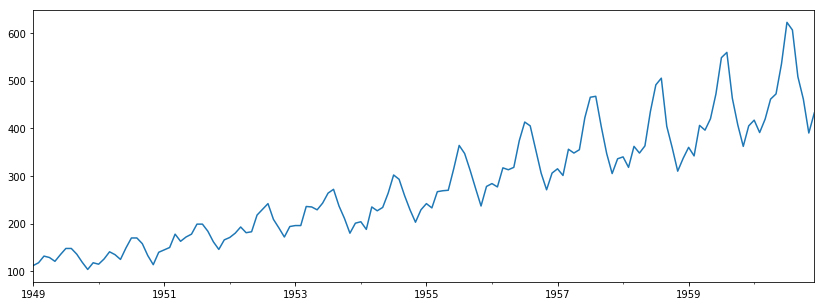We note a couple of visuall indications of non-stationarity:

- The mean of the process appears to be increasing as time increases;
- The variance of the process (i.e. the fluctuations) appear to be smaller at the beginning and much larger at the end of the time series
- The monthly data appears to exhibit a seasonality pattern

We can view this seasonality by plotting each year as a separate plot.

Note that since we have a time series variable

In :
airpass.index

Out:
DatetimeIndex(['1949-01-01', '1949-02-01', '1949-03-01', '1949-04-01',
'1949-05-01', '1949-06-01', '1949-07-01', '1949-08-01',
'1949-09-01', '1949-10-01',
...
'1960-03-01', '1960-04-01', '1960-05-01', '1960-06-01',
'1960-07-01', '1960-08-01', '1960-09-01', '1960-10-01',
'1960-11-01', '1960-12-01'],
dtype='datetime64[ns]', length=144, freq='MS')

We can extract its year and month

In :
print(airpass.index.year)
print(airpass.index.month)

Int64Index([1949, 1949, 1949, 1949, 1949, 1949, 1949, 1949, 1949, 1949,
...
1960, 1960, 1960, 1960, 1960, 1960, 1960, 1960, 1960, 1960],
dtype='int64', length=144)
Int64Index([ 1,  2,  3,  4,  5,  6,  7,  8,  9, 10,
...
3,  4,  5,  6,  7,  8,  9, 10, 11, 12],
dtype='int64', length=144)


which we can use to transpose the data:

In :
data_to_plot = pd.pivot_table(airpass.to_frame(), index = airpass.index.month, columns = airpass.index.year)

Out:
value
1949 1950 1951 1952 1953 1954 1955 1956 1957 1958 1959 1960
1 112 115 145 171 196 204 242 284 315 340 360 417
2 118 126 150 180 196 188 233 277 301 318 342 391
3 132 141 178 193 236 235 267 317 356 362 406 419
4 129 135 163 181 235 227 269 313 348 348 396 461
5 121 125 172 183 229 234 270 318 355 363 420 472

and plot the seasonal plots

In :
fig = plt.figure(figsize = (20, 8))
plt.show()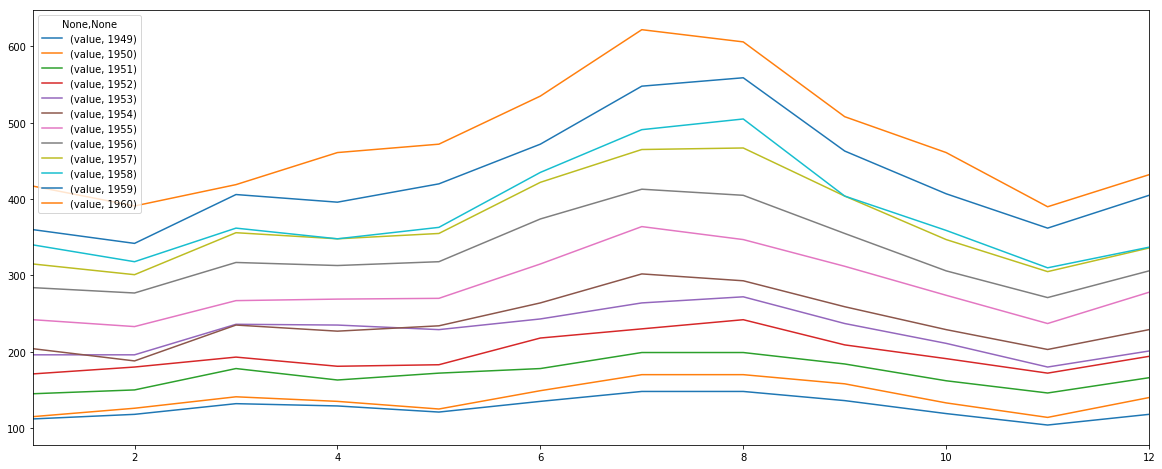We see that there appears to be an increase in passengers between June and August yeach year, with the least amount of airline passengers in February and November of each year.

This appears to be a deterministic (i.e. non-random and repeating) pattern.

Overall, we have that the series is not stationary.

### (1.2) Autocorrelation¶

One way to check for autocorrelation is to examine the ACF and PACF plots, which we can do using our own tsdisplay function:

In :
tsdisplay(airpass)

c:\users\andrius\appdata\local\programs\python\python37\lib\site-packages\matplotlib\axes\_axes.py:6521: MatplotlibDeprecationWarning:
The 'normed' kwarg was deprecated in Matplotlib 2.1 and will be removed in 3.1. Use 'density' instead.
alternative="'density'", removal="3.1")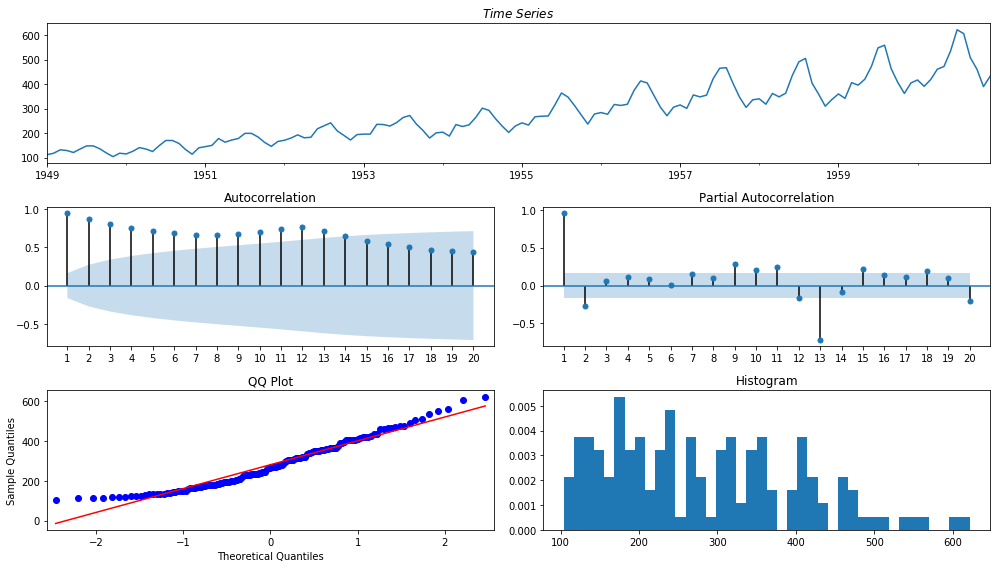To test for significant autocorrelation with the Ljung-Box test. We will again opt to use our won function as it is a bit more convenient:

In :
tsdiag(airpass)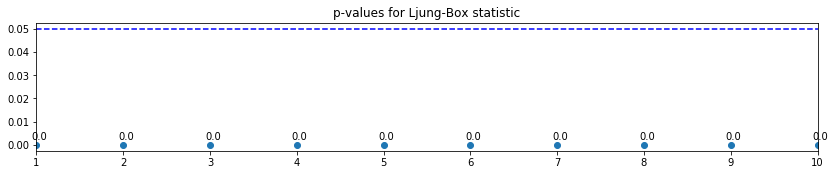Out:
1 2 3 4 5 6 7 8 9 10
Ljung-Box: X-squared 1.321415e+02 2.456462e+02 3.426748e+02 4.277387e+02 5.047966e+02 5.756019e+02 6.430386e+02 7.094845e+02 7.795912e+02 8.570686e+02
Ljung-Box: p-value 1.393231e-30 4.556318e-54 5.751088e-74 2.817731e-91 7.360195e-107 4.264008e-121 1.305463e-134 6.496271e-148 5.249370e-162 1.100789e-177
Box-Pierce: X-squared 1.294263e+02 2.398212e+02 3.335270e+02 4.150951e+02 4.884584e+02 5.553839e+02 6.186636e+02 6.805584e+02 7.453831e+02 8.164925e+02
Box-Pierce: p-value 5.471060e-30 8.384679e-53 5.499710e-72 1.522155e-88 2.473624e-103 9.752966e-117 2.327567e-129 1.095987e-141 1.203020e-154 5.870025e-169

Since all the p-values are less than the 0.05 critical value, we reject the null hypothesis of no autocorrelation and conclude that the series is autocorrelated.

We also see from the ACF plot that:

• the ACF decayse very slowly - this indicates a trend effect.
• the ACF plot exhibits a larger correlation at around every $12^{th}$ lag (at lags 12, 24, 36, etc. the correlation spykes). This is an indication that there is a seasonal (or cyclic) component in our data.

Since we have monthly data, where every $12^{th}$ lag exhibits a stronger autocorrelation, the seasonal period is $d = 12$. Usually the seasonal period equal the frequency of the time series.

### (1.3) Decomposition Type and data transformation¶

We again state that the variance of the process (i.e. the fluctuations) appear to be smaller at the beginning and much larger at the end of the time series. This indicated that our series may be multiplicative: $Y_t = T_t \cdot S_t \cdot E_t$.

Since in practice it is much easier to deal with additive series: $Y_t = T_t + S_t + E_t$, we will transform the data by taking the logarithms:

In :
log_passengers = np.log(airpass)

In :
tsdisplay(log_passengers)

c:\users\andrius\appdata\local\programs\python\python37\lib\site-packages\matplotlib\axes\_axes.py:6521: MatplotlibDeprecationWarning:
The 'normed' kwarg was deprecated in Matplotlib 2.1 and will be removed in 3.1. Use 'density' instead.
alternative="'density'", removal="3.1")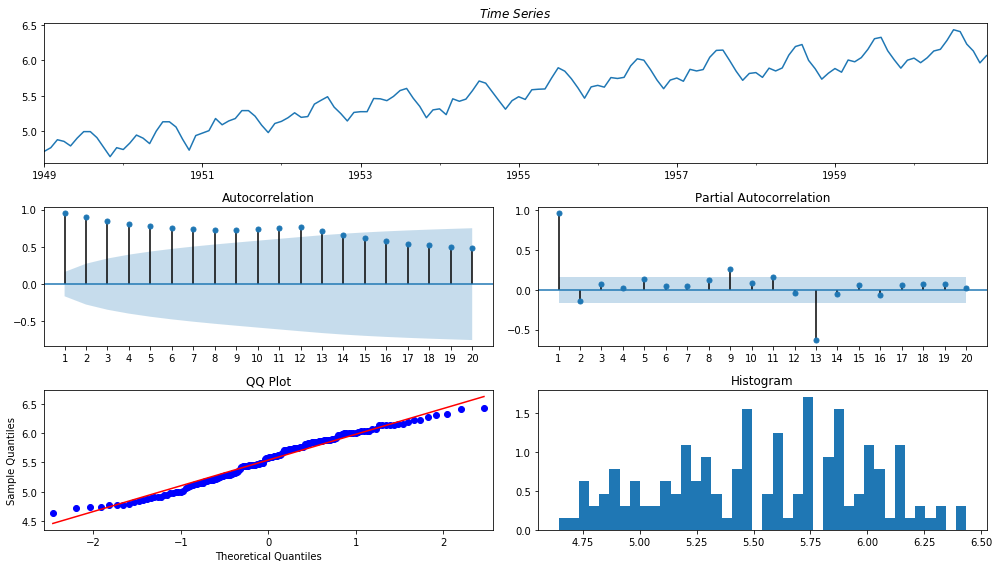The series appears to now have a constant variance - the fluctuations at the beginning appear to be similar to those at the end of the time series.

Note that the minimum value in our series is a positive value:

In :
print(airpass.min())

104


hence we can take the logarithms of our data.

## (2.1) Time Series Decomposition WITHOUT Forecasts¶

We will begin by examining a simple decomposition method based on averaging (more specifically we select the amount of values that we will use for the averaging and "move" the averaging window based on time $t$). This is known as the moving-average method for decomposition.

We will begin by estimating the trend component of the series.

### Trend Decomposition via Moving Average¶

We will provide an example of the moving-average method for decomposition.

If we select the length of the moving average as an odd number, for example $l = 3$, then:

• The two-sided moving-average:
$$\widehat{T}_t = \dfrac{Y_{t-1} + Y_t + Y_{t+1}}{3}$$
• The one-sided moving-average:
$$\widehat{T}_t = \dfrac{Y_{t-2} + Y_{t-1} + Y_t}{3}$$

are calculated for integer times $t$.

If the time series contains a seasonal component and we want to averate it out, the length of the moving average must be equal to the seasonal frequency (for a monthly series, the length $l = 12$). However, there is a slight hurdle - suppose, our times series begin in January ($t = 1$) and we average up to December ($t = 12$). This average corresponds to a time $t = 6.5$ - a time between June and July.

When we come to estimate seasonal effects, we need a moving-average at integer times. This can be achieved by averaging the average of January to December and the average of February ($t = 2$) to January ($t = 13$). This average of the two moving averages corresponds to $t = 7$ and the process is called centering.

Thus, the trend at time $t$ can be estimated by the centered moving average:

• Two-sided averaging: \begin{aligned} \widehat{T}_t &= \dfrac{(Y_{t-6} +...+ Y_{t+5})/12 + (Y_{t-5} +...+ Y_{t+6})/12}{2} = \dfrac{(1/2)Y_{t-6} + Y_{t-5}...+ Y_{t+5}+ (1/2)Y_{t+6}}{12} \end{aligned}

• One-sided averaging: \begin{aligned} \widehat{T}_t &= \dfrac{(Y_{t-12} +...+ Y_{t-1})/12 + (Y_{t-11} +...+ Y_{t})/12}{2} = \dfrac{(1/2)Y_{t-12} + Y_{t-11}...+ Y_{t-1}+ (1/2)Y_{t}}{12} \end{aligned}

Note that the weights are different for the first and last elements used in the averaging. we can use the statsmodels.tsa.seasonal.seasonal_decompose to automatically decompose our series.

We will begin with a two-sided decomposition:

In :
decomposition_1  = smt.seasonal.seasonal_decompose(log_passengers, model = "additive", freq = 12, two_sided = True)
trend_1 = decomposition_1.trend


We can plot this decomposition alongside the time series

In :
plt.figure(figsize = (15, 6))
log_passengers.plot(label = "log of Airpassangers")
trend_1.plot(label = "Two-sided decomposition of the trend")
plt.legend()
plt.show()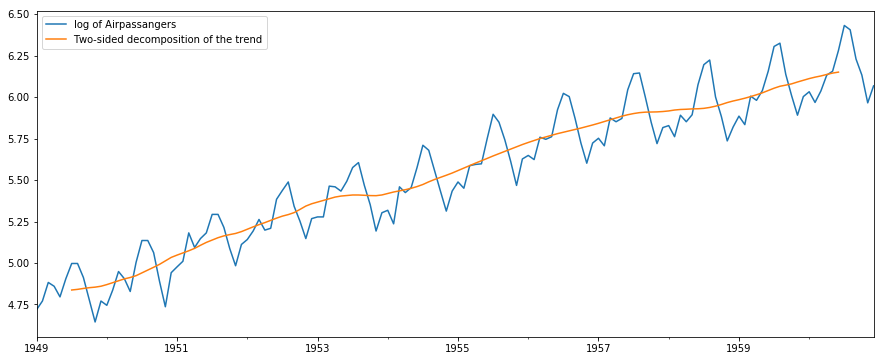Since we used a two-sided decomposition, we cannot calculate the trend for the first and last 6 months of our monthly time series.

On the other hand, if we specify a one-sided decomposition, then we will not be able to calculate the trend for the first 12 months:

In :
decomposition_2  = smt.seasonal.seasonal_decompose(log_passengers, model = "additive", freq = 12, two_sided = False)
trend_2 = decomposition_2.trend

In :
plt.figure(figsize = (15, 6))
log_passengers.plot(label = "log of Airpassangers")
trend_2.plot(label = "One-sided decomposition of the trend")
plt.legend()
plt.show()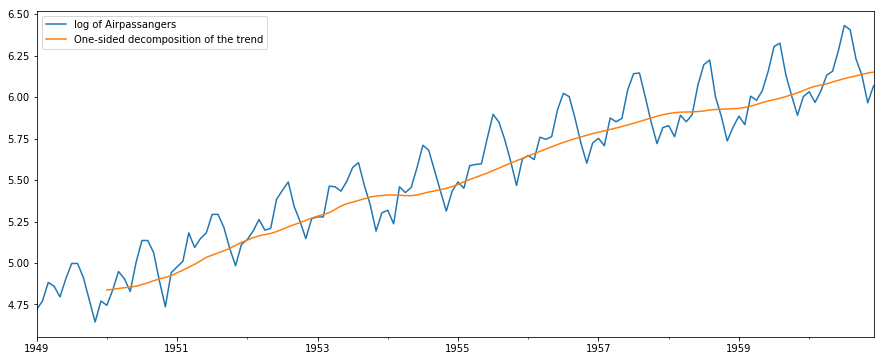We would like to stress the importance of correctly selecting the period of the series. If we select a lower period value, then we will begin to capture the seasonal part with our trend filter.

For example, selecting the moving average length $l = 3$, or selecting too large of a period frequency length, say $l = 19$, yields:

In :
decomposition_3  = smt.seasonal.seasonal_decompose(log_passengers, model = "additive", freq = 3, two_sided = False)
trend_3 = decomposition_3.trend

plt.figure(figsize = (10, 6))
log_passengers.plot(label = "log of Airpassangers")
trend_3.plot(label = "One-sided decomposition of the trend with d = 3")
trend_2.plot(label = "One-sided decomposition of the trend woth period d = 12")
plt.legend()
plt.show()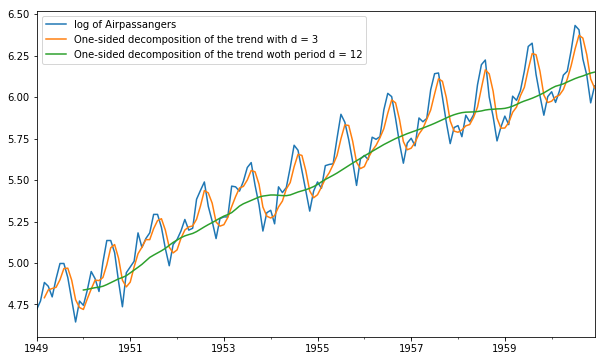This can be explained by looking at the formulas for the moving averagedecomposition - this is because we are using fewer observations around time $t$. So, our estimated trend values would be closer to the overall series value. However, in doing so, we are not estimating the trend component correctly. As such, it is important to correctly determine whether your data exhibits a seasonal component and if so - to correctly determine the seasonal frequency.

Furthermore, specifying too large of a period frequency length, say $l = 19$ yields:

In :
decomposition_4  = smt.seasonal.seasonal_decompose(log_passengers, model = "additive", freq = 24, two_sided = False)
trend_4 = decomposition_4.trend

plt.figure(figsize = (10, 6))
log_passengers.plot(label = "log of Airpassangers")
trend_4.plot(label = "One-sided decomposition of the trend with period d = 24")
trend_2.plot(label = "One-sided decomposition of the trend woth period d = 12")
plt.legend()
plt.show()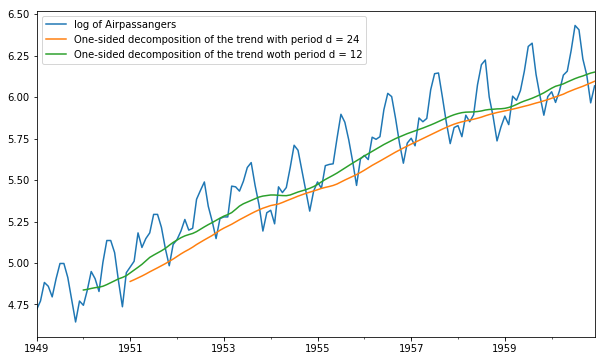Notice that too large of a period may not capture the trend as accurately. Again, as we take more values, which cover more than one period, we calculate the average value over more than one period, hence our trend estimate is not as accurate.

We could also try to specify our own function to decompose the trend only:

In :
import math as math

def moving_average_decompose(y, l = 12):
tmp_index = y.index
#Time Series:
y = np.array(y)
#Trend Component:
t_two_side = pd.np.zeros(y.size)
t_one_side = pd.np.zeros(y.size)
tmp_val = 0
#Loop through each time point:
for j in range(0, y.size):
###########################################################
# The length is even - use centered averaging:
if l % 2 == 0:
# The centered averaging method weights - see the formula example with l = 12.
tmp_weights = np.concatenate([np.array([1 / (l * 2)]),
np.repeat(1 / l, l - 1),
np.array([1 / (l * 2)])])
#Two-sided
if j + 1 <= l / 2 or j + 1 > y.size - l / 2:
# We do not have enough data to the left or right of the series to calculate trend at time t = j:
t_two_side[j] = np.nan
else:
tmp_val = y[int(j - (l / 2)):int(j + (l / 2 + 1))] * tmp_weights
t_two_side[j] = tmp_val.sum()
#One-sided
if j + 1 <= l:
t_one_side[j] = np.nan
else:
tmp_val = y[int(j - l):int(j + 1)] * tmp_weights
t_one_side[j] = tmp_val.sum()
###########################################################
# The length is odd:
else:
#For the non-centered averaging the weights are simply the mean, i.e. the length l.
#tmp_weights = np.repeat(1 / l, l)
#Two-sided
if j + 1 <= math.floor(l / 2) or j + 1 > y.size - math.floor(l / 2):
# We do not have enough data to the left or right of the series to calculate trend at time t = j:
t_two_side[j] = np.nan
else:
tmp_val = y[int(j - math.floor(l / 2)):int(j + (math.floor(l / 2) + 1))]
t_two_side[j] = tmp_val.mean()

#One-sided
if j * 2 <= l:
t_one_side[j] = np.nan
else:
tmp_val = y[int(j - l + 1):int(j + 1)]
t_one_side[j] = tmp_val.mean()
#Return the same time series:
t_one_side = pd.Series(t_one_side, name = "One-Sided MA with length = " + str(l))
t_one_side.index = tmp_index
t_two_side = pd.Series(t_two_side, name = "Two-Sided MA with length = " + str(l))
t_two_side.index = tmp_index

return {"Two-sided": t_two_side, "One-sided": t_one_side}


Here we have applied the formulas for calculating the moving average for both odd and even length of the period.

We can test our function as follows:

In :
decomposition_custom_1 = moving_average_decompose(log_passengers)
decomposition_custom_2 = moving_average_decompose(log_passengers, l = 3)
decomposition_custom_3 = moving_average_decompose(log_passengers, l = 24)

plt.figure(figsize = (20, 10))
log_passengers.plot()
decomposition_custom_1["One-sided"].plot()
decomposition_custom_1["Two-sided"].plot()
decomposition_custom_2["Two-sided"].plot()
decomposition_custom_3["One-sided"].plot()
plt.legend()
plt.show()